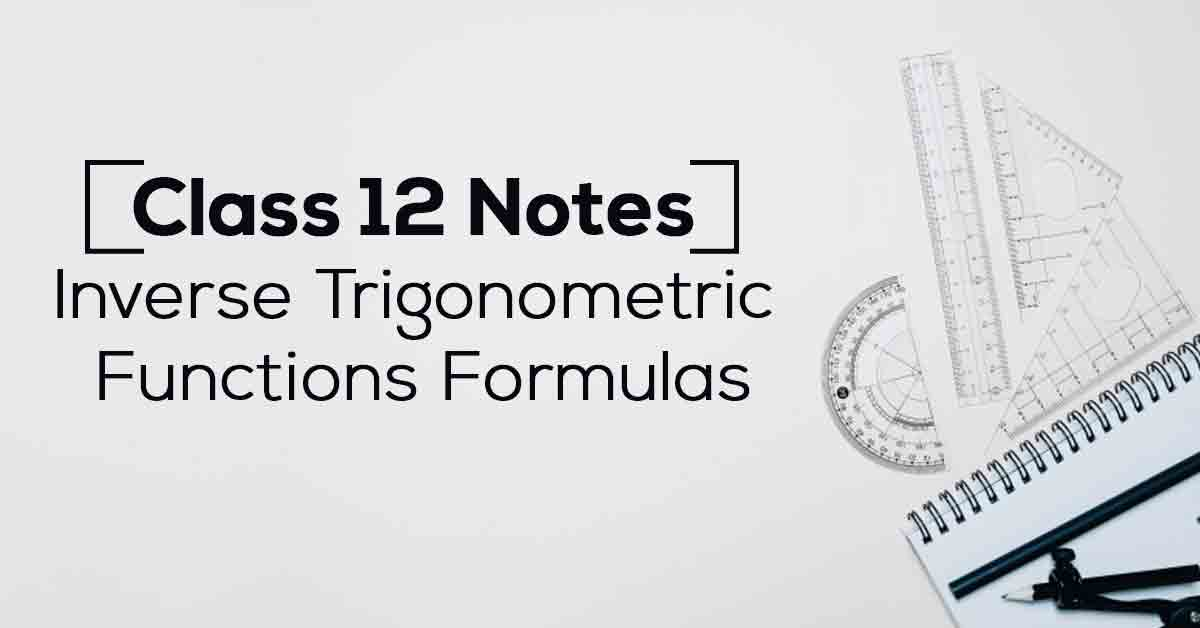Inverse Trigonometric Functions Class 12 Formulas and Notes | Vidyakul
×

# Inverse Trigonometric Functions Class 12 Formulas and Notes## Class 12 Maths Chapter 2 Inverse Trigonometric Functions Class 12 Formulas & Notes – PDF Download

Inverse function. Inverse of a function ‘f ‘ exists, if the function is one-one and onto, i.e, bijective. Since trigonometric functions are many-one over their domains, we restrict their domains and co-domains in order to make them one-one and onto and then find their inverse. Know More about these in Inverse Trigonometric Functions Class 12 Formulas and Notes List.

The topics and sub-topics covered in Inverse Trigonometric Functions Class 12 Formulas and Notes are:

2.1 Introduction

2.2 Basic Concepts

2.3 Properties of Inverse Trigonometric Functions.

Download the FREE PDF of Inverse Trigonometric Functions Class 12 Formulas and Notes and start your preparation with Vidyakul!